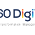### Research Paper Summary - A robust Modification on K Nearest Neighbor Classifier

Original Paper :A Modification of K Nearest Neighbor Classifier
Authors : Hamid Parvin, Hoseinali Alizadeh, Befrouz Minati

### K - Nearest Neighbor Classifier

KNN is one of the simplest classification algorithm that is used widely across the problems where there is little or no idea of prior distribution of the data. More about KNN can be learn from this or this.
Here is a naive implementation of KNN from scratch. Take a look at it as it will improve your understanding about the internals of the algorithm.
A cup of fact: You can tweet KNN's implementation from scratch. Here is one such implementation( not by me )

### Modified K - Nearest Neighbor Classifier

The main problem in KNN is that not all points or nearest neighbors have same importance. Also, when the dimensions of the data increases, the accuracy of KNN decreases ( curse of dimensionality).
In this paper, the author has proposed a modification to tackle above problems by some pre-computation of dataset and getting the weights(or validity as called by the author) of all the point which is later used for prediction.
How to compute the validity :
1. The validity of each point is computed according to it's H neighbors.
2. Validity of a point is the probability of occurrence of same labeled points in it's neighborhood, where neighborhood is considered by H closest points.
3. In simple terms, validity of a point is the total number of points, among nearest  H points, that have same label as the point in consideration.
4. The above count is divided be H to normalize it between 0 and 1.
Formula stated:
Validity(x) = (sum( similarity(x, N(i)) )) / H
where N(i) is the i'th neighbor of x and
similarity(x, y) = 1 if x.label == y.label else 0
How to use the above calculated weights for classification:
Rather than simple counting/majority voting system used in KNN, this modification uses a weight factor(i.e. validity) to assign importance to the points.
The weight of a point is usually a decreasing function of distance of sample from unknown sample. So, here comes another multiplication factor d(e).
d(e,x) = 1.0/(euclidean_distance(e,x)+ alpha), alpha=0.5 usually and works as smoothing factor.
Using the above factor, the final weight W(y) of a point y is calculated as
W(y) = validity(y) * d(y,x) where x is the unknown point to be classified.
Finally, for classification, score of each label is calculated, using K (not H, used above) nearest neighbors, and then the label with maximum score is assigned to the unknown point x.
The final scores are calculated as:
score(label=L) = sum of W(y) of points y having label = L, which are in K nearest neighbors of x.
prediction(x) = label L_dash having maximum score.
Benefits:
1. Overcome the weakness of distance based weighing methods in cases of outliers.
2. Assign greater importance to more 'validated' sample than to unstable samples.

Experimental results of using this method can be read from the paper itself.

1.Amazing Article ! I would like to thank you for the efforts you had made for writing this awesome article. This article inspired me to read more. keep it up.
Correlation vs Covariance
Simple Linear Regression
data science interview questions
KNN Algorithm

2.Amazing Article ! I would like to thank you for the efforts you had made for writing this awesome article. This article inspired me to read more. keep it up.
Correlation vs Covariance
Simple Linear Regression
data science interview questions
KNN Algorithm

3.Amazing Article ! I would like to thank you for the efforts you had made for writing this awesome article. This article inspired me to read more. keep it up.
Correlation vs Covariance
Simple Linear Regression
data science interview questions
KNN Algorithm
Logistic Regression explained

4.I am happy to visit here. Thanks to my friend who told me about this webpage, this blog is really awesome.

5.Hello Guest,
Awesome post, really helpful, Thanks for sharing...

6.Extremely useful information which you have shared here. This is a great way to enhance knowledge for us, and also helpful for us. Thankful to you for sharing an article like compiler design research papers.

7.Great post I would like to thank you for the efforts you have made in writing this interesting and knowledgeable article. data analytics course in mysore